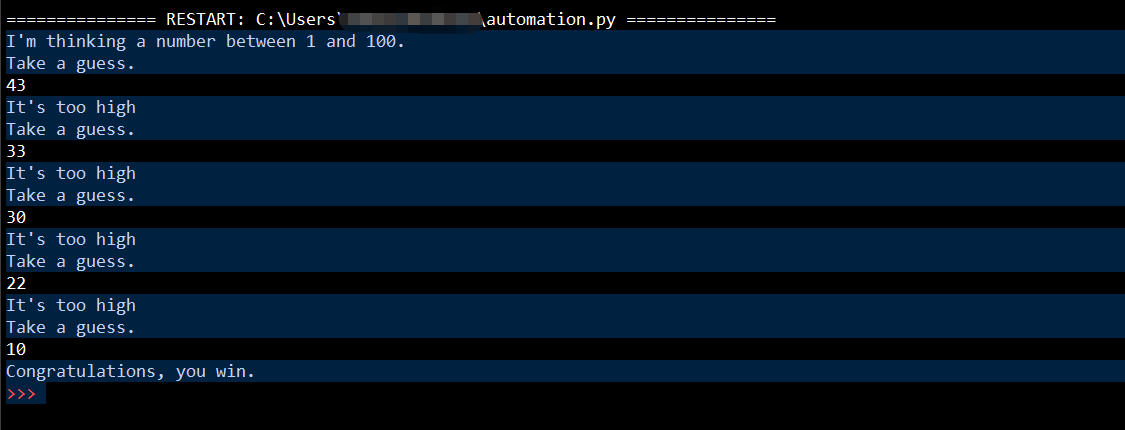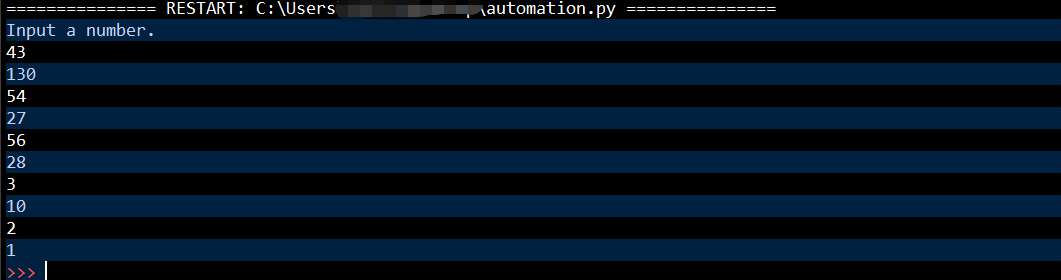# 关于我们

## 新闻公告

< 返回

### Python如何实现猜数字小程序

#### 猜数字

```'''

'''
guessNumber = random.randint(1,100)
print("I'm thinking a number between 1 and 100.")

while True:
print('Take a guess.')

guess = int(input())

if guess > guessNumber:
print("It's too high")
elif guess < guessNumber:
print("It's to low")
else:
print("Congratulations, you win.")
break```
• 结果#### Collatz序列

• 代码

```#Collatz序列
def collatz(number):
if (number % 2 == 0):
return number / 2
else:
return number * 3 + 1

print('Input a number.')

while True:
global number1
try:
number1 = int(input())
except ValueError:
continue
if collatz(number1) != 1:
print(int(collatz(number1)))
else:
print(int(collatz(number1)))
break```
• 结果/template/Home/Dawn/PC/Static